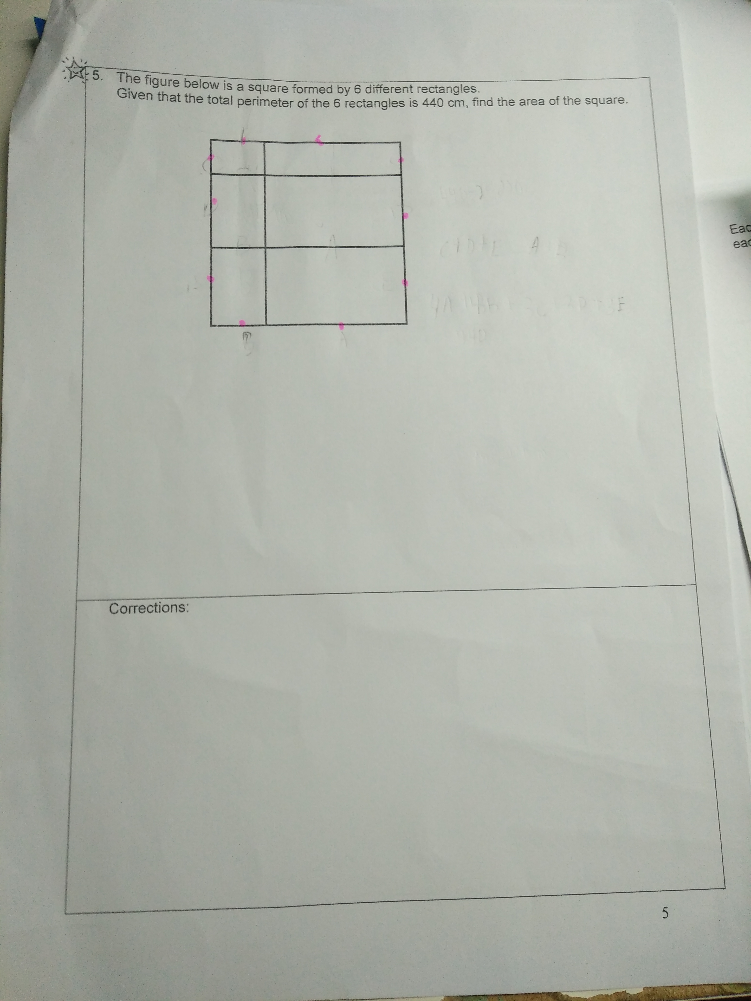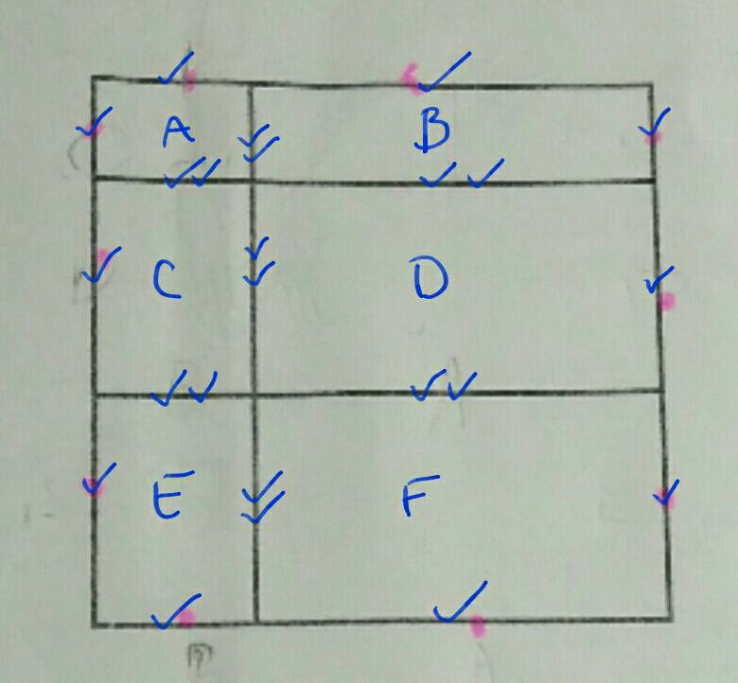# QuestionHi can someone help me with the following question above? Thank you so much!:)We add up the sides (line segments) of the 6 rectangles A, B, C, D, E, F to find the total perimeter

For each line segment, the number of ticks is the number of times it is added

We can easily see that all the line segments add up 10 times of the side of the square

So the side of the square = 440 cm ÷ 10 = 44 cm

The area of the square = 44 cm x 44 cm = 1936 cm2

Hope it helps 🙂